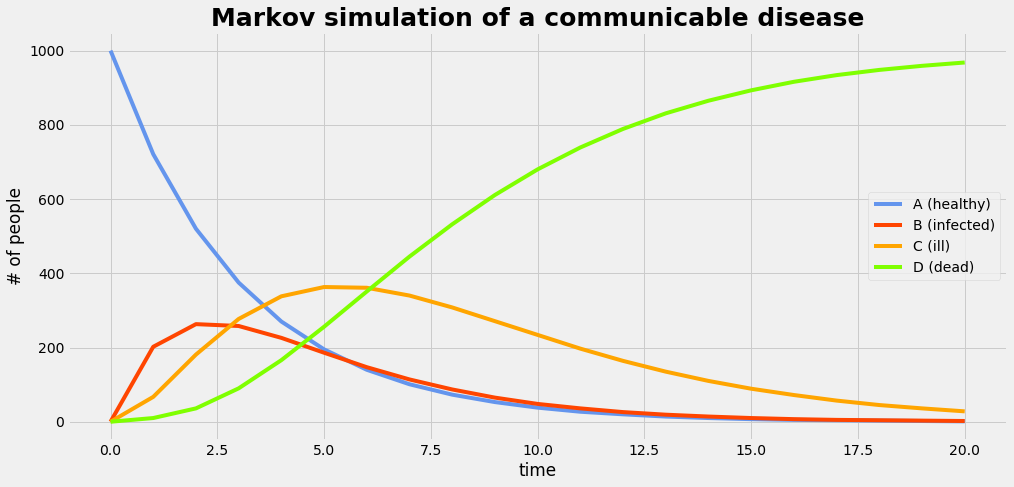.

.

.

.

.

# HealthyNumerics

HealthPoliticsEconomics | Quant Analytics | Numerics

# NumericalStats: WHY to switch from a decision tree to a Markov model

import numpy as np
import pandas as pd
from scipy import stats
from matplotlib import pyplot as plt
import matplotlib.colors as mclr


## Why a change could be indicated

The decision tree is a simple form of decision model. But there are also limitions of the decision tree evident:

• a formal aspect is that the tree format becomes rapidly unwiedly when a combination of several options have to be mapped
• with regard to content the elapse of time is not explicit in decision trees. Many chronic diseases such as diabetes, ischaemic heart disease or some forms of cancer have a recuring-remitting pattern over a period of many years. If a longer time horizon has to be adopted, several features may become necessary to be modelled:
• continuing risk of recurrence
• competing risk of death as the cohort ages
• other clinical developments

The Markov model is an approach to handel added modelling options. The key structure of a markov model is:

• it is structured around disease states
• it is driven by a set of possible transitions between the disease states
• it can be run over a series of time periods which gives an insight over the temporal evolution of the disease states
• costs may be included in parallel
• the modelled transistions probabilities may change over time too, so that changing conditions may be included

We give in the follwing a basic example of a Markov model that illustrates the temporal evolution of a communicable disease in a population.

$$\begin{equation} \begin{array}{rcl} \textrm{Markov matrix} \; \mathbf{M} &=& \left[\begin{matrix}0.721 & 0.202 & 0.067 & 0.010 \\ 0.000 & 0.581 & 0.407 & 0.012 \\ 0.000 & 0.000 & 0.750 & 0.250 \\ 0.000 & 0.000 & 0.000 & 1.000 \end{matrix}\right] \\ \textrm{Start vector} \;\; \mathbf{p}_0 &=& \left[\begin{matrix}1 \\ 0 \\0 \\0\end{matrix}\right]\\ Repeat:\\ \textrm{Time step} \;\; \mathbf{p}_1 &=& \mathbf{M}^T \cdot \mathbf{p}_0 \\ \textrm{Iteration} \;\; \mathbf{p}_0 &:=& \mathbf{p}_1 \end{array} \end{equation}$$
d1## Set up the Markov system

M_states = np.array(['A (healthy)', 'B (infected)', 'C (ill)', 'D (dead)'])
MM = np.array([[0.721,  0.202,  0.067,  0.010],
[0.000,  0.581,  0.407,  0.012],
[0.000,  0.000,  0.750,  0.250],
[0.000,  0.000,  0.000,  1.000] ])
dmm = pd.DataFrame(MM, columns=M_states, index=M_states); dmm

A (healthy) B (infected) C (ill) D (dead)
A (healthy) 0.721 0.202 0.067 0.010
B (infected) 0.000 0.581 0.407 0.012
C (ill) 0.000 0.000 0.750 0.250
D (dead) 0.000 0.000 0.000 1.000

## Run the Markov simulation

We run the simulation with a population of 1000 members. Note that simulation part has 7 lines of code only:

nRuns = 21
m_result = np.zeros((nRuns,len(M_states)))
v0 = np.array([1, 0, 0, 0])*1000
for ir in range(nRuns):
m_result[ir,:] = v0
v0 = np.dot(MM.T,v0)
dmr = pd.DataFrame(np.rint(m_result).astype(int), columns=M_states)


## Plot the result

plot_gr02(dmr,'time','# of people','Markov simulation of a communicable disease'); dmrA (healthy) B (infected) C (ill) D (dead)
0 1000 0 0 0
1 721 202 67 10
2 520 263 181 36
3 375 258 277 90
4 270 226 338 166
5 195 186 363 256
6 140 147 361 351
7 101 114 340 445
8 73 87 308 532
9 53 65 271 611
10 38 48 234 680
11 27 36 197 739
12 20 26 164 789
13 14 19 135 831
14 10 14 110 865
15 7 10 89 893
16 5 7 72 916
17 4 5 57 934
18 3 4 45 948
19 2 3 36 959
20 1 2 28 968
import graphviz as gv
def plotMarkovModel():
d1 = gv.Digraph(format='png',engine='dot')
c = ['cornflowerblue','orangered','orange','chartreuse']
d1.node('A','A (healthy)', style='filled', color=c)
d1.node('B','B (infected)',style='filled', color=c)
d1.node('C','C (ill)',     style='filled', color=c)
d1.node('D','D (dead)',    style='filled', color=c)
d1.edge('A','A',label='0.721'); d1.edge('A','B',label='0.202'); d1.edge('A','C',label='0.067'); d1.edge('A','D',label='0.010')
d1.edge('B','B',label='0.581');                                 d1.edge('B','C',label='0.407'); d1.edge('B','D',label='0.012')
d1.edge('C','C',label='0.750');                                                                 d1.edge('C','D',label='0.250')
d1.edge('D','D',label='1.000');
return d1
d1 = plotMarkovModel()
d1.render('img/mamo1', view=True)

def plot_gr02(DF,xLabel,yLabel,grTitel):
with plt.style.context('fivethirtyeight'):
fig = plt.figure(figsize=(15,7)) ;
ax1 = fig.add_subplot(111);
#DF.plot(ax = plt.gca())
colors = ['cornflowerblue','orangered','orange','chartreuse']
DF.plot(ax = ax1, style='-', color=colors)
plt.xlabel(xLabel);  plt.ylabel(yLabel);
plt.title(grTitel, fontsize=25, fontweight='bold');
plt.show()